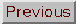### PERTURBATION THEORY

Adapted from P. Coles, 1999, The Routledge Critical Dictionary of the New Cosmology, Routledge Inc., New York. Reprinted with the author's permission. To order this book click here: http://www.routledge-ny.com/books.cfm?isbn=0415923549

Often in physics we have to apply relatively simple physical laws to extremely complicated situations. Although it is straightforward to write down the equations necessary to calculate what a given physical system will do, it may be difficult to solve these equations unless the situation has a particular symmetry, or if some aspects of the problem can be neglected. A good example lies in the construction of cosmological models using general relativity. The Einstein equations are extremely complicated, and no general solutions are available. However, if we assume that the Universe is completely homogeneous and isotropic (i.e. if we invoke the cosmological principle), then the special symmetry implied by the Robertson-Walker metric drastically simplifies the problem. We ends up with the Friedmann models, and only the relatively simple Friedman equation to solve.

But the Universe is not exactly homogeneous and isotropic now, even if the cosmic microwave background radiation suggests that it must have been so earlier on (see large-scale structure). One of the most important questions asked by cosmologists is how this structure came about. In order to make a theory of structure formation, surely we need to solve the Einstein equations for the general case of an inhomogeneous and anisotropic universe? To be precise, the answer to this question is `yes', but to be reasonably accurate the answer is `no'. Even though our Universe is not exactly homogeneous, it is almost so. If we calculate the expected departures from the Robertson-Walker metric for all the mass concentrations we know about, we find them to be small - about one part in a hundred thousand. So we need solutions of the Einstein equations that describe an almost but not quite homogeneous universe. For this we need a model which is almost a Friedmann model, but not quite.

The mathematical technique for generating solutions of equations that are almost the same as solutions you already know is called perturbation theory, and it is used in many branches of physics other than cosmology. The basic idea can be illustrated as follows. Suppose we have to calculate (1.0001)9 without using a calculator. This problem can be thought of as being almost like calculating 19, because the quantity in brackets is not far from 1; and 19 is just 1. Suppose that we represent the extra 0.0001 we have to deal with by the symbol. The problem now is to calculate the product of nine terms 1 +: (1 +) (1 +)....(1 +). Now imagine multiplying out this expression term by term. Since there are nine brackets each with two terms (a 1 and an), there are 29 = 512 combinations altogether - quite a task. The first term would be a 1, which is obtained by multiplying all the 1's in all the brackets. This would be the biggest term, because there are no other terms bigger than 1 and all the terms containingare much smaller. If we multiplied thein the first bracket by the 1's in all the others, we would get. By taking oneand eight 1's in every possible way from the nine brackets we would get nine terms altogether, all of which are. Now, any other terms made in more complicated ways that this, like five 1's and four's, would result in powers of(in this case4). But becauseis smaller than 1, all these terms are much smaller thanitself, and very much smaller than 1. It should therefore be a good approximation just to keep the nine terms in whichappears on its own, and ignore terms that contain2 or3 or higher powers of. This suggests that we can write, approximately,

(1 +)91 + 9Going back to our original problem, we can put= 0.0001, from which we find that the approximate answer to be 1.0009. In fact, the right answer is 1.000 900 36. So our approximation of taking only the lowest-order correction () to a known solution (19 = 1) works very well in this case.

The way to exploit this idea in cosmology is to begin with the equations that describe a Friedmann model for which the Robertson-Walker metric (which we denote here by g) holds. We know how to handle these equations, and can solve them exactly. The we write the equations again, not in terms of g but in terms of some other quantity g' = g + h where h is a small correction likein the above example - in other words, a perturbation. If h is small, we can neglect all the terms of order higher than h and obtain a relatively simple equation for how h evolves. This is the approach used to study the growth of small primordial density fluctuations in the expanding Universe.

Of course, the approach breaks down when the small correction becomes not so small. The method used above does not work at all well for (1.1)9, for example. In the study of structure formation by means of the Jeans instability, the fluctuations gradually grow with time until they become large. We then have to abandon perturbation methods and resort to another approach. In the example above, we have to reach for a calculator. In cosmology, the final nonlinear stages have to be handled in a similar brute-force way, by running N-body simulations.

FURTHER READING:

Coles, P. and Lucchin, F., Cosmology: The Origin and Evolution of Cosmic Structure (John Wiley, Chichester, 1995), Part 3.